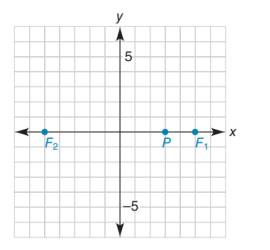Chapter 10.1, Problem 44E### Elementary Geometry for College St...

6th Edition
Daniel C. Alexander + 1 other
ISBN: 9781285195698

#### Solutions

Chapter
Section### Elementary Geometry for College St...

6th Edition
Daniel C. Alexander + 1 other
ISBN: 9781285195698
Textbook Problem
1 views

# By definition, a hyperbola is the locus of points whose positive difference of distances from two fixed points F 1 and F 2 (called foci) is constant. In the grid provided, find points whose difference of distances from points F 1 5, 0 and F 2 - 5, 0 is 6. That is, locate some points for which P F 1 - P F 2 = 6 or P F 2 - P F 1 = 6 ; point P 3, 0 is one such point. Then sketch the hyperbola.To determine

To sketch:

The hyperbola using the given data.

Explanation

The general equation of the hyperbola is shown below.

x2a2-y2b2=1

Vertices ±a, 0.

Foci ±c, 0

Here c2=a2+b2

The foci of the hyperbola is F15, 0 and F2-5, 0.

Since, foci ±c, 0

Hence, c=5

In the problem, it is given that one point is 3, 0.

Vertices ±a, 0

The other point is -3, 0.

Since PF1-PF2=6

2a=6

Dividing by 2,

2a2=62

From this,

a=3

Using the values of a and c, we have to find b

### Still sussing out bartleby?

Check out a sample textbook solution.

See a sample solution

#### The Solution to Your Study Problems

Bartleby provides explanations to thousands of textbook problems written by our experts, many with advanced degrees!

Get Started